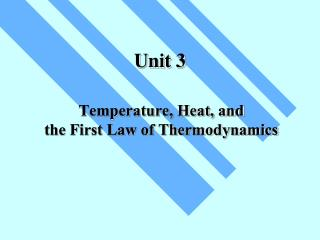DownloadDownload PresentationUnit 3

# Unit 3

Download Presentation## Unit 3

- - - - - - - - - - - - - - - - - - - - - - - - - - - E N D - - - - - - - - - - - - - - - - - - - - - - - - - - -
##### Presentation Transcript

1. Unit 3 Temperature, Heat, and the First Law of Thermodynamics

2. You heat an object It gets hot Heat Capacity (cal/K, or J/K) Specific Heat (cal/g · K, or J/kg·K) Absorption of Heat (add heat to it) (temperature increases)

3. By definition of heat, the specific heat ofwateris c = 1cal/g · K higher than most other substance

4. The thermal energy required for the transition: Latent Heat (cal/g, or J/kg) Latent Heat During some phase transitions (i.e. ice - water), heating does not lead to increase of temperature until the transition is completed

5. The work doneBYthe gas during the volumechange fromVitoVf Work Done During Volume Change Consider a gas cylinder of piston areaA, gaspressurep, and gas volumeV The gas expands, the piston moves byds, andthe volume changes fromVtoV+dV=V+A·ds The work doneBYthe gas: dW=F·ds=A·p·ds=p·dV

6. is the area under the curve in thep-V diagramrepresenting a path fromVitoVf SameViand Vf, different path different area different work P-V Diagram (Pay attention to the direction of the path!!) Close cycle W = enclosed area

7. The First Law of Thermodynamics: difference of internal energy heat added to the system work done by the system The First Law of Thermodynamics For given initial and final points, Q - Wis thesame for all paths.

8. Infinitesimal process or work done ON the system or The First Law of Thermodynamics The changeofinternal energyispath independent Theinternal energyis astate function

9. Cyclic process:∆Eint = 0Q = W = Area enclosed by the cycle • Free expansion: Q = 0, W = 0∆Eint = 0 • Isovolumetric process: W = 0∆Eint = Q • Adiabatic process:Q = 0∆Eint = -W

10. Heat Transfer Mechanisms • Conduction • through the materials • Convection • through the movement of a heated substance • Radiation • through emission of electromagnetic field

11. Temperature gradient Rate of heat transfer Thermal conductivity (W/m K) Cross-section Conduction Exchange of kinetic energy • Between molecules or atoms (insulators) • By “free electrons” (metals)

12. Convection Heat transfer by the movement of a heated substance. • Natural convection Result from difference in density • Forced convection The heated substance is forced to move

13. Temperature Stefan-Boltzmann constant s =5.67051 x 10-8 W/m2K4 Power of radiation Area Emissivity Absorption: Net absorption: Radiation Radiation:

14. HRW 54E(5th ed.).A 150 g copper bowl contains 220 g of water, both at 20.0˚C. A very hot 300 g copper cylinder is dropped into the water, causing the water to boil, with 5.00 g being converted to steam. The final temperature of the system is 100˚C. (a) How much heat was transferred to the water? (b) How much to the bowl? (c) What was the original temperature of the cylinder? (a) The heat transferred to the water of mass ml is: Qw= cwmw∆T + LVms = (1 cal/gC˚)(220g)(100˚C-20.0˚C)+(539 cal/g)(5.00 g) = 20.3 kcal

15. (c) Let it be Ti, then -Qw- Qb = ccmc(Tf-Ti) HRW 54E(5th ed.).A 150 g copper bowl contains 220 g of water, both at 20.0˚C. A very hot 300 g copper cylinder is dropped into the water, causing the water to boil, with 5.00 g being converted to steam. The final temperature of the system is 100˚C. (a) How much heat was transferred to the water? (b) How much to the bowl? (c) What was the original temperature of the cylinder? (b) The heat transferred to the bowl is: Qb= cbmb∆T = (0.0923 cal/gC˚)(150g)(100˚C-20.0˚C)= 1.11 kcal

16. HRW 75E(5th ed.).Gas within a chamber passes through the cycle shown in Fig. 19-37. Determine the net heat added to the system during process CA if the heat QAB added during process AB is 20.0 J, no heat is transferred during process BC, and the net work dome during the cycle is 15.0 J. Since the process is a complete cycle (beginning and ending in the same thermodynamic state), ∆Eint = 0 and Q = W, QAB + QBC + QCA = W QCA = W- QAB - QBC = 15.0 J - 20.0 J - 0 = -5.0 J 5.0 J of energy leaves the gas in the form of heat.

17. HRW 84E(5th ed.).A cylindrical copper rod of length 1.2 m and cross-sectional area 4.8 cm2 is insulated to prevent heat loss through its surface. The ends are maintained at a temperature difference of 100˚C by having one end in a water-ice mixture and the other in boiling water and steam. (a) Find the rate at which heat is conducted along the rod. (b) Find the rate at which ice melts at the cold end. (a) The rate at which the heat is conducted along the rod (b) The rate at which the ice melts is